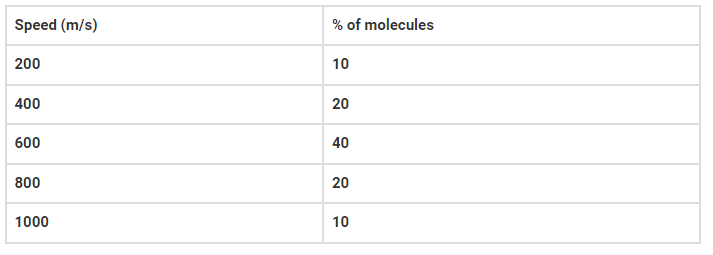# Consider an ideal gas with`
Question:

Consider an ideal gas with the following distribution of speeds(a) calculate Vrms and hence T (m = 3.0 × 10-26 kg)

(b) if all the molecules with speed 1000 m/s escape from the system, calculate new Vrms and hence T

Solution:

(a) T = 296 K

(b) T = 248.04 K